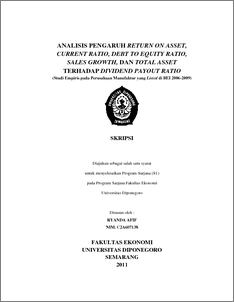# ANALISIS PENGARUH RETURN ON ASSET, CURRENT RATIO, DEBT TO EQUITY RATIO, SALES GROWTH, DAN TOTAL ASSET TERHADAP DIVIDEND PAYOUT RATIO (Studi Empiris pada Perusahaan Manufaktur yang Listed di BEI 2006-2009)

AFIF, Ryanda and CHABACHIB, Chabachib (2011) ANALISIS PENGARUH RETURN ON ASSET, CURRENT RATIO, DEBT TO EQUITY RATIO, SALES GROWTH, DAN TOTAL ASSET TERHADAP DIVIDEND PAYOUT RATIO (Studi Empiris pada Perusahaan Manufaktur yang Listed di BEI 2006-2009). Undergraduate thesis, Universitas Diponegoro.Preview
PDF - Published Version
356Kb

## Abstract

This research was conducted to examine the influence of the company's financial performance through the Return On Assets (ROA), Current Ratio (CR), Debt to Equity Ratio (DER), Sales Growth, and Total Assets of Dividend Payout Ratio (DPR) manufacturing companies listed in Indonesia Stock Exchange (BEI) in the period 2006 to 2009. The problem of this research is due to the contradiction between the theory with the facts about the influence Return On Assets (ROA), Current Ratio (CR), Debt to Equity Ratio (DER), Sales Growth, and Total Assets of Dividend Payout-year observation period 2006 to 2009. The sampling of this study using purposive sampling method, a sample of 11 companies that match the criteria specified by the study period 2006-2009, data obtained from the Indonesian Capital Markets Directory and the Annual Report of the company. The analytical method used is multiple regression with a quadratic equation of the smallest and hypothesis test using the t-statistic for testing the partial regression coefficient and F-statistics to test the effect of simultaneously. In addition, previously also tests the classical assumption that includes testing normality, multicollinearity test, test Heteroskidastity, and the autocorrelation test. The results showed that there were no deviation with classical assumptions, this suggests that the available data has been fulfilling the requirements for use of multiple linear regression model. The resulting regression equation DPR = 41.314 + 1.146 ROA - 5.323 CR - 4.859 DER -20 451 GROWTH - 0.012 ASSET + e. From the analysis shows partial results that ROA variable has positive and significant impact on the Parliament. Variable CR, DER, and Growth significantly and negatively related to the House of Representatives. Asset variable has a negative but not significant to the House of Representatives. Simultaneously , Current Ratio, Debt to Equit Ratio, Sales Growth, and Total Asset and significant effect on DPR. Then the results of regression estimates indicate predictive ability of the 5 independent variables of DPR by 29% while the rest 71% influenced by other factors outside the model that has not been included in this analysis.

Item Type: Thesis (Undergraduate) Return On Asset (ROA), Current Asset (CR), Debt to Equity Ratio (DER), Sales Growth, Total Asset , Deviden Payout Ratio (DPR) Return On Asset (ROA), Current Asset (CR), Debt to Equity Ratio (DER), Sales Growth, Total Asset , Deviden Payout Ratio (DPR) H Social Sciences > H Social Sciences (General) Faculty of Economics and Business > Department of Management 29276 Mr. Perpustakaan Fakultas Ekonomi 06 Sep 2011 13:13 06 Sep 2011 13:13

Repository Staff Only: item control page